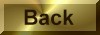Practice Problem 6

Write a balanced equation for the reaction between the permanganate ion and hydrogen peroxide in a basic solution to form manganese dioxide and oxygen.

 MnO4-(aq) + H2O2(aq)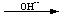MnO2(s) + O2(g)

Solution

STEP 1: Write a skeleton equation for the reaction.

MnO4- + H2O2MnO2 + O2

STEP 2: Assign oxidation numbers to atoms on both sides of the equation.
 MnO4- + H2O2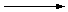MnO2 + O2 +7 -2 +1 -1 +4 -2 0

STEP 3: Determine which atoms are oxidized and which are reduced.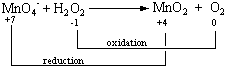STEP 4: Divide the reaction into oxidation and reduction half-reactions and balance these half-reactions. This reaction can be divided into the following half-reactions.

 Reduction: MnO4-MnO2 +7 +4
 Oxidation: H2O2O2 -1 0

Let's start by balancing the reduction half-reaction. It takes three electrons to reduce manganese from the +7 to the +4 oxidation state.

 Reduction: MnO4- + 3 e-MnO2

We now try to balance either the number of atoms or the charge on both sides of the equation. Because the reaction is run in basic solution, we can add either OH- ions or H2O molecules to either side of the equation, as needed. The key to deciding which side of the equation gets each of these reagents is simple: The only way to balance the net charge of -4 on the left side of the equation is to add four OH- ions to the products.

 Reduction: MnO4- + 3 e-MnO2 + 4 OH-

We can then balance the number of hydrogen and oxygen atoms by adding two H2O molecules to the reactants.

 Reduction: MnO4- + 3 e- + 2 H2OMnO2 + 4 OH-

We now turn to the oxidation half-reaction. Two electrons are lost when hydrogen peroxide is oxidized to form O2 molecules.

 Oxidation: H2O2O2 + 2 e-

We can balance the charge on this half-reaction by adding a pair of OH- ions to the reactants.

 Oxidation: H2O2 + 2 OH-O2 + 2 e-

The only way to balance the number of hydrogen and oxygen atoms is to add two H2O molecules to the products,
 Oxidation: H2O2 + 2 OH-O2 + 2 H2O + 2 e-

as shown in the figure below.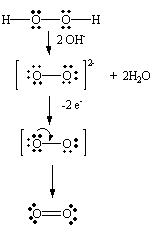STEP 5: Combine the two half-reactions so that electrons are neither created nor destroyed. Two electrons are given off during the oxidation half-reaction and three electrons are consumed in the reduction half-reaction. We can therefore combine these half-reactions by using the lowest common multiple of 2 and 3.
 2(MnO4- + 3 e- + 2 H2OMnO2 + 4 OH-) + 3(H2O2 + 2 OH-O2 + 2 H2O + 2 e-) ________________________________________________________ 2 MnO4- + 3 H2O2 + 6 OH- + 4 H2O2 MnO2 + 3 O2 + 8 OH- + 6 H2O

STEP 6: Balance the remainder of the equation by inspection, if necessary. The simplest balanced equation is obtained by subtracting four H2O molecules and six OH- ions from each side of the equation derived in the previous step.

2 MnO4-(aq) + 3 H2O2(aq)2 MnO2(s) + 3 O2(g) + 2 OH-(aq) + 2 H2O(l)

Note that the ratio of moles of MnO4- to moles of H2O2 consumed is different in acidic and basic solutions. This difference results from the fact that MnO4- is reduced all the way to Mn2+ in acid, but the reaction stops at MnO2 in base.